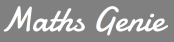# Maths

#### Curriculum Intent - Maths:

Learning mathematics at Liskeard School & Community College is an inter-connected journey, encouraging students to link areas of mathematics which may not be immediately obvious. We support students to think, reason and explain, aiming for them to apply their fluency skills, with conviction, to reason and problem solve. Articulating and justifying ideas is at the heart of our maths classroom culture. We teach essential skills which enable our students to be successful in their chosen next steps. Through our enthusiasm and love of mathematics, we inspire confidence, develop resilience and ensure inclusivity for all.

## #TEAMMATHS Knowledge Organiserhttps://www.onmaths.com/

This is great for exam practise. You can complete full exam papers or topic specific exam questions online. These are marked as you go through the paper so you can see your progress and GCSE grade as you work through the questions.https://www.sparxmaths.uk/

You know about this one, but have you used the Independent Learning section? Simply type in the topic area that you wish to revise and it will generate questions for you to practise.https://www.mymaths.co.uk/

You’ll be familiar with MyMaths. You can work through lessons and complete online homework using the login details:https://www.mathsgenie.co.uk/gcse.html

This website is great for practising graded exam questions. There are exam questions, worked solutions and videos too.

## Curriculum Overview Maths

Autumn Spring Summer

Year 7

Sequences 1

Algebra 1

Number 1

FDP 1

Calculating 1

FDP 2

Number 2

FDP 3

Geometry 1

Probability 1

Geometry 2

Year 8

Ratio 1

Ratio 2

FDP 4

Graphs 1

Statistics 1

Probability 2

Algebra 2

Sequences 2

Calculating 2

FDP 5

Number 3

Geometry 3

Shape 1

Statistics 2

Geometry 4

Year 9

Number 4

Algebra 3

Graphs 2

Shape 2

FDP 6

Calculating 3

Geometry 5

Shape 3

Geometry 6

Probability 3

Year 10 Foundation

Number 5 (F)

Calculating 4 (F)

FDP 7 (F)

Number 6 (F)

Algebra 4 (F)

Ratio 3 (F)

Sequences 3 (F)

Algebra 5 (F)

Shape 4 (F)

Geometry 7 (F)

Graphs 3 (F)

Algebra 6 (F)

Probability 4 (F)

Statistics 3 (F)

Year 10 Higher

Number 5 (H)

Algebra 4 (H)

Geometry 7 (H)

Geometry 8 (H)

Ratio 3 (H)

Sequences 3 (H)

Algebra 5 (H)

Shape 5 (H)

Geometry 9 (H)

FDP 7 (H)

Algebra 6 (H)

Graphs 3 (H)

Probability 4 (H)

Statistics 3 (H)

Graphs 4 (H)

Geometry 10 (H)

Year 11 Foundation

FDP 8 (F)

Geometry 8 (F)

Number 7 (F)

Algebra 7 (F)

Geometry 9 (F)

Algebra 8 (F)

Ratio 4 (F)

Sequences 4 (F)

Shape 4 (F)

Graphs 4 (F)

Statistics 4 (F)

Geometry 10 (F)

Geometry 11 (F)

Revision

Year 11 Higher

Geometry 11 (H)

Number 6 (H)

Algebra 7 (H)

Shape 6 (H)

Algebra 8 (H)

Ratio 4 (H)

Sequences 4 (H)

Algebra 9 (H)

Graphs 5 (H)

Statistics 4 (H)

Ratio 5 (H)

Geometry 12 (H)

Revision

Year 12

Polynomials

Graphs, Inequalities and transformations

Binomials

Trigonometry

Differentiation

Integration

Straight lines and circles

Exponentials and logarithms

Vectors

Proof

Sampling and data interpretation

Kinematics

Probability and statistical distributions

Hypothesis testing

Forces

Year 13

Trigonometry and circular measure

Further differentiation

Further Integration

Binomials, sequences and series

Functions and transformations

Parametric Equations

Further Trigonometry
Partial fractions

Differential equations

Numerical methods

Further Probability

Statistical Distributions

Kinematics in two dimensions

Equilibrium and resolving

Statics and dynamics

Statistical hypothesis testing

Moments